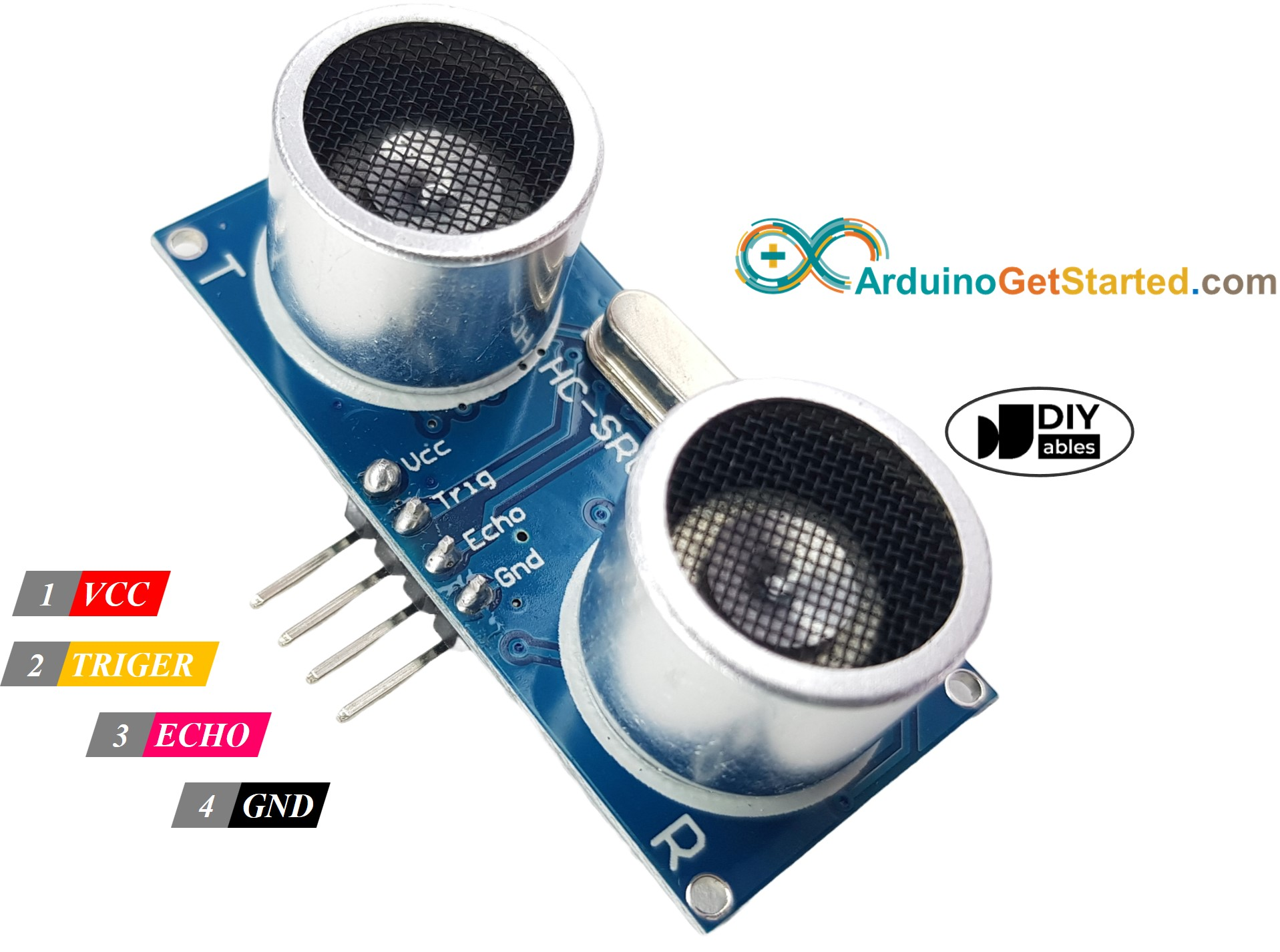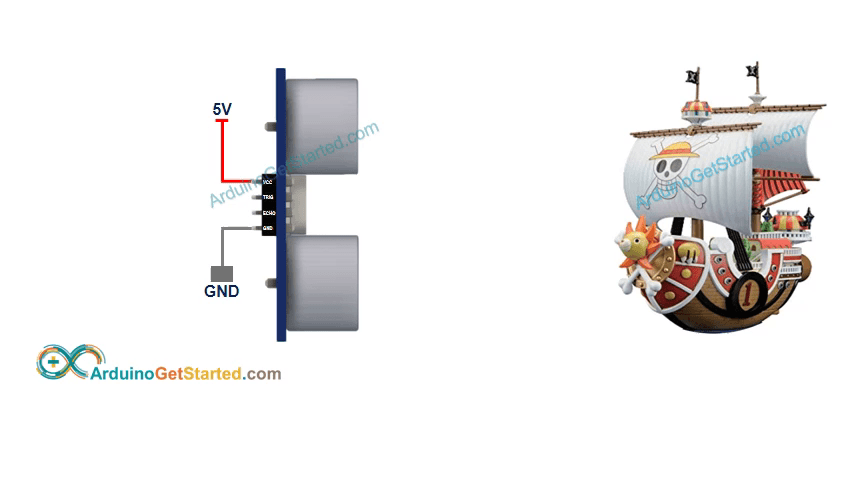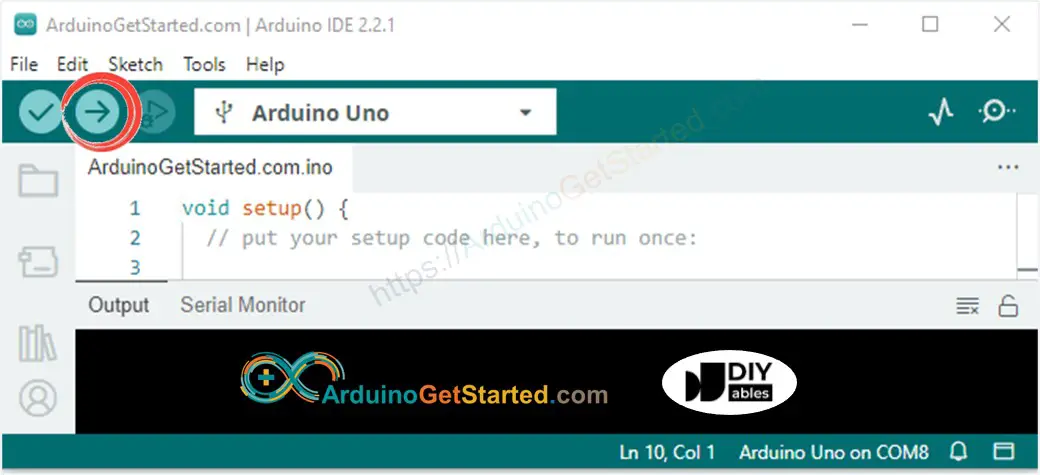# Arduino - Ultrasonic Sensor

## Hardware Required

Please note: These are affiliate links. If you buy the components through these links, We may get a commission at no extra cost to you. We appreciate it.

Ultrasonic sensor HC-SR04 is used to measure the distance to an object by using ultrasonic waves.

### Pinout

The ultrasonic sensor HC-SR04 includes four pins:

• VCC pin needs to be connected to VCC (5V)
• GND pin needs to be connected to GND (0V)
• TRIG pin this pin receives the control signal (pulse) from Arduino.
• ECHO pin this pin sends a signal (pulse) to Arduino. Arduino measures the duration of pulse to calculate distance.
•### How It Works

This section is the in-depth knowledge. DON'T worry if you don't understand. Ignore this section if it overloads you, and come back in another day. Keep reading the next sections.
1. Micro-controller: generates a 10-microsecond pulse on the TRIG pin.
2. The ultrasonic sensor automatically emits the ultrasonic waves.
3. The ultrasonic wave is reflected after hitting an obstacle.
4. The ultrasonic sensor:
• Detects the reflected ultrasonic wave.
• Measures the travel time of the ultrasonic wave.
5. Ultrasonic sensor: generates a pulse to the ECHO pin. The duration of the pulse is equal to the travel time of the ultrasonic wave.
6. Micro-controller measures the pulse duration in the ECHO pin, and then calculate the distance between sensor and obstacle.
7.### How to Get Distance From Ultrasonic Sensor

To get distance from the ultrasonic sensor, we only need to do two steps (1 and 6 on How It Works part)

• Generates a 10-microsecond pulse on TRIG pin
• Measures the pulse duration in ECHO pin, and then calculate the distance between sensor and obstacle

#### Distance Calculation

We have:

• The travel time of the ultrasonic wave (µs): travel_time = pulse_duration
• The speed of the ultrasonic wave: speed = SPEED_OF_SOUND = 340 m/s = 0.034 cm/µs

So:

• The travel distance of the ultrasonic wave (cm): travel_distance = speed × travel_time = 0.034 × pulse_duration
• The distance between sensor and obstacle (cm): distance = travel_distance / 2 = 0.034 × pulse_duration / 2 = 0.017 × pulse_duration

### Arduino - Ultrasonic Sensor

Arduino's pins can generate a 10-microsecond pulse and measure the pulse duration. Therefore, we can get the distance from the ultrasonic sensor by using two Arduino's pins:

• One pin is connected to TRIG PIN to generate 10µs pulse to TRIG pin of the sensor
• Another pin is connected to ECHO PIN measure pulse from the sensor

## Wiring DiagramImage is developed using Fritzing

## How To Program

• How to generate a 10-microsecond pulse in Arduino's pin, for example, pin 9:
digitalWrite(9, HIGH); delayMicroseconds(10); digitalWrite(9, LOW);
• How to measures the pulse duration (µs) in Arduino's pin, for example, pin 8:
duration_us = pulseIn(8, HIGH);
• How to calculate distance (cm):
distance_cm = 0.017 * duration_us;

## Arduino Code

/* * Created by ArduinoGetStarted, https://arduinogetstarted.com * * Arduino - Ultrasonic Sensor HC-SR04 * * Wiring: Ultrasonic Sensor -> Arduino: * - VCC -> 5VDC * - TRIG -> Pin 9 * - ECHO -> Pin 8 * - GND -> GND * * Tutorial is available here: https://arduinogetstarted.com/tutorials/arduino-ultrasonic-sensor.php */ int trigPin = 9; // TRIG pin int echoPin = 8; // ECHO pin float duration_us, distance_cm; void setup() { // begin serial port Serial.begin (9600); // configure the trigger pin to output mode pinMode(trigPin, OUTPUT); // configure the echo pin to input mode pinMode(echoPin, INPUT); } void loop() { // generate 10-microsecond pulse to TRIG pin digitalWrite(trigPin, HIGH); delayMicroseconds(10); digitalWrite(trigPin, LOW); // measure duration of pulse from ECHO pin duration_us = pulseIn(echoPin, HIGH); // calculate the distance distance_cm = 0.017 * duration_us; // print the value to Serial Monitor Serial.print("distance: "); Serial.print(distance_cm); Serial.println(" cm"); delay(500); }

### Quick Steps

• Copy the above code and open with Arduino IDE
• Click Upload button on Arduino IDE to upload code to Arduino
•• Open Serial Monitor
• Move your hand in front of ultrasonic sensor
• See the distance from the sensor to your hand on Serial Monitor
• COM6
distance: 29.4 cm distance: 27.6 cm distance: 26.9 cm distance: 17.4 cm distance: 16.9 cm distance: 14.3 cm distance: 15.6 cm distance: 13.1 cm
Autoscroll Show timestamp
Clear output
9600 baud
Newline

### Code Explanation

Read the line-by-line explanation in comment lines of code!

## Challenge Yourself

Use ultrasonic sensor to do one of the following projects:

• Collision avoidance for RC car.
• Detecting the fullness of dustbin.
• Monitoring level of the dustbin.
• Automatically open/close the dustbin. Hint: Refer to Arduino - Servo Motor .

Some manufacturers provide the ultrasonic sensor that has 3 pins. TRIG signal and ECHO signal are in the same pin. In this case, we need to use only one Arduino's pin for both purposes: generating a pulse to the sensor and measuring pulse from the sensor.

## Alternative Ultrasonic Sensors

The above code also works with the following ultrasonic sensor:

## Ultrasonic Sensor on Commercial Products

• Otto DIY Robot Builder Kit

## Ultrasonic Sensor Applications

• Collision Avoidance
• Fullness Detection
• Level Measurement
• Proximity Detection